Chapter 4 Class 11 Mathematical Induction

Class 11
Important Questions for exams Class 11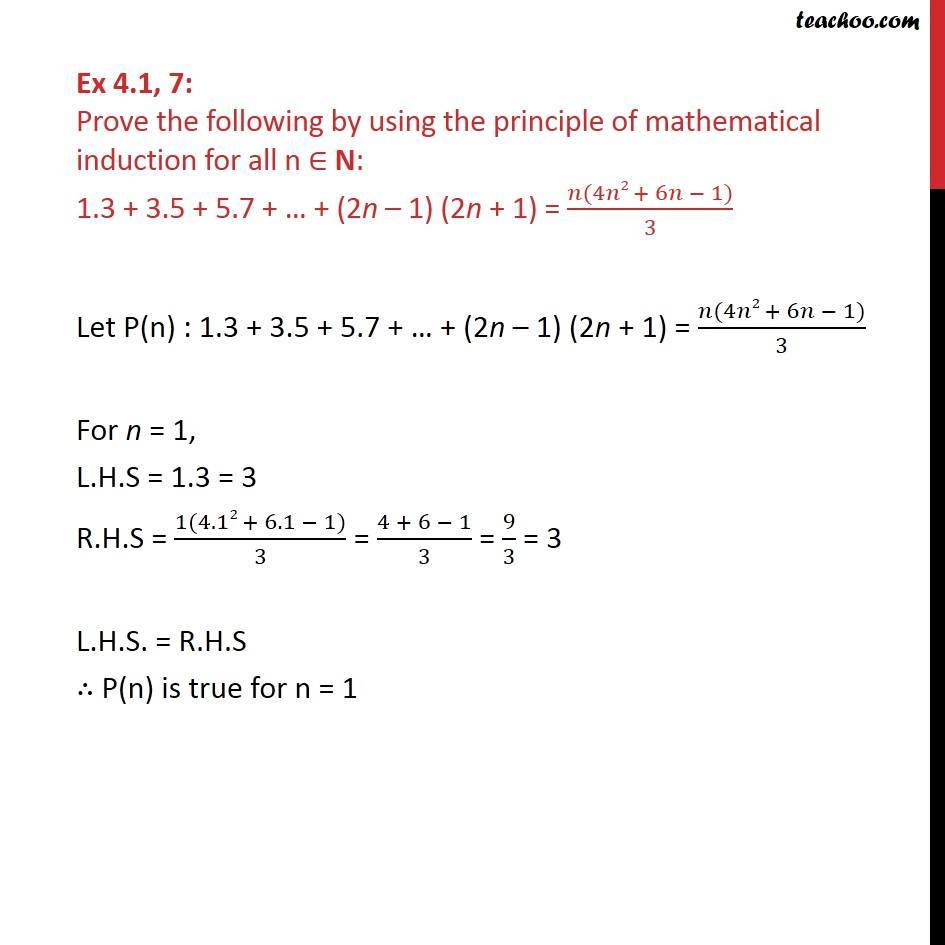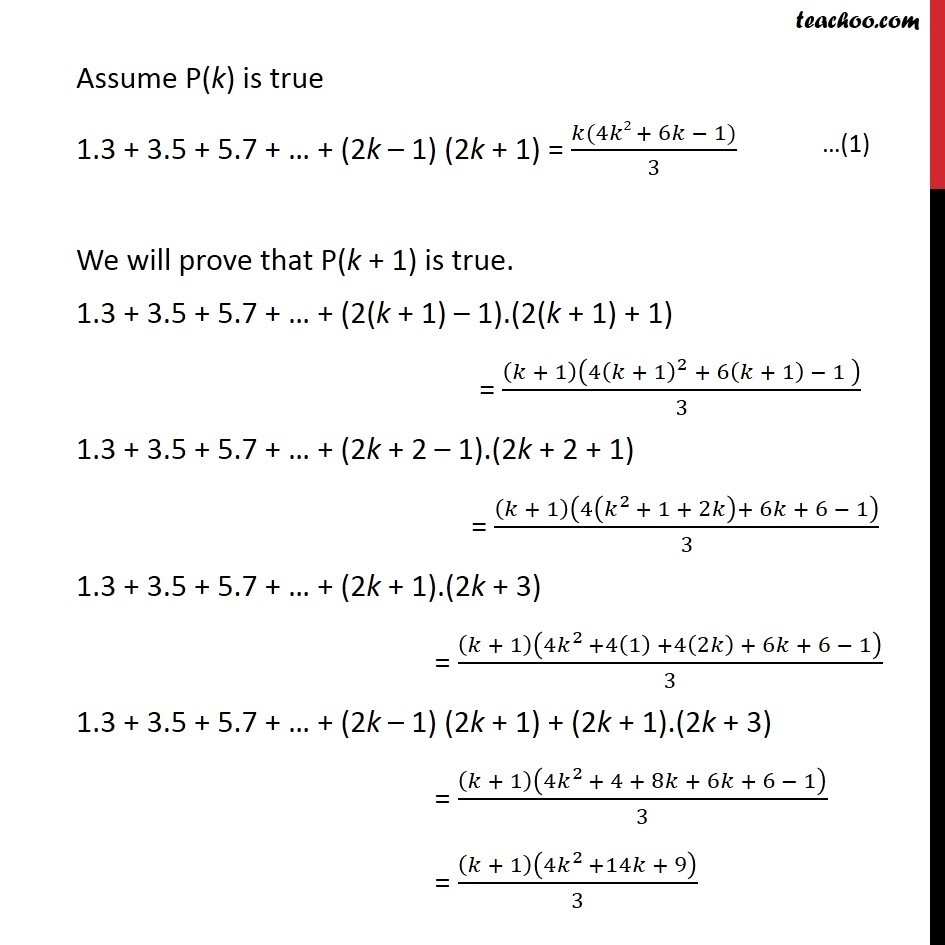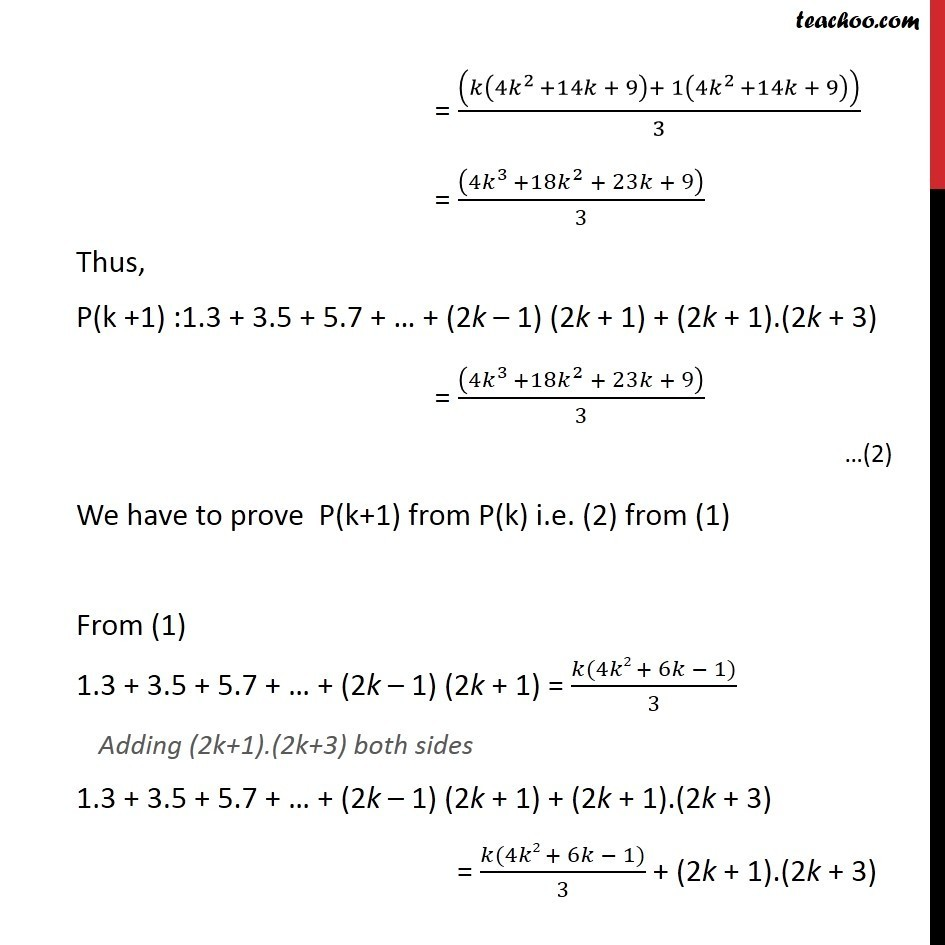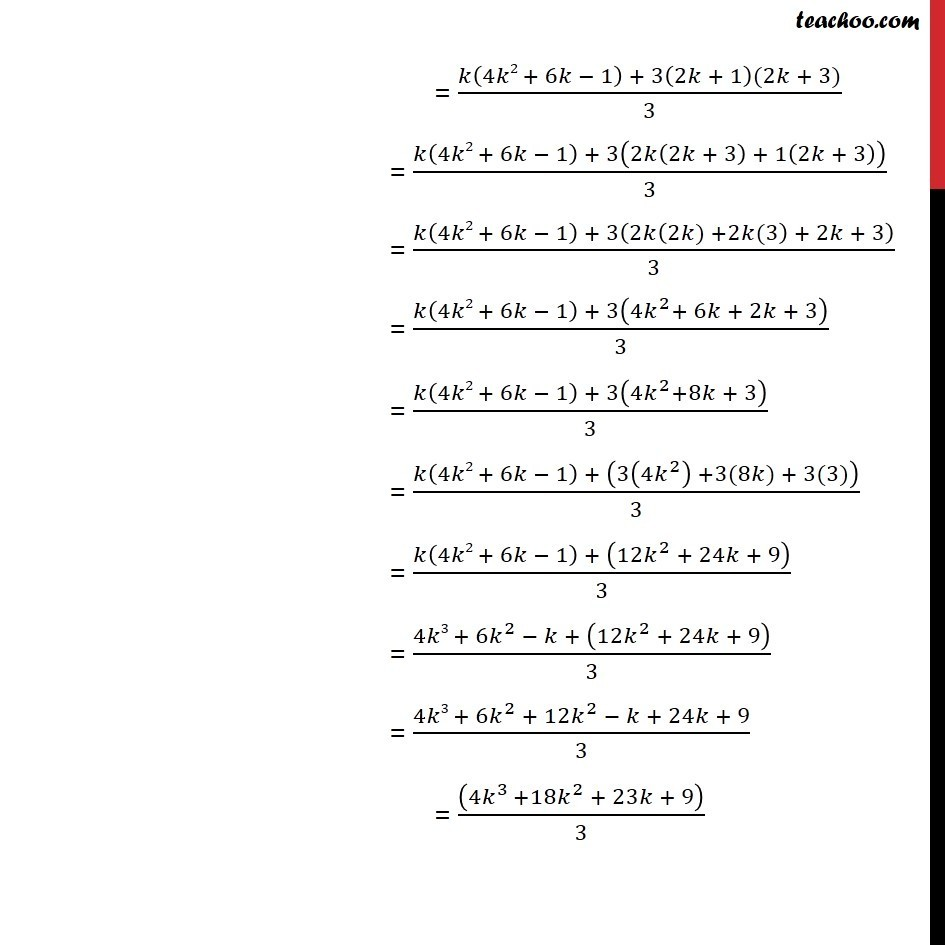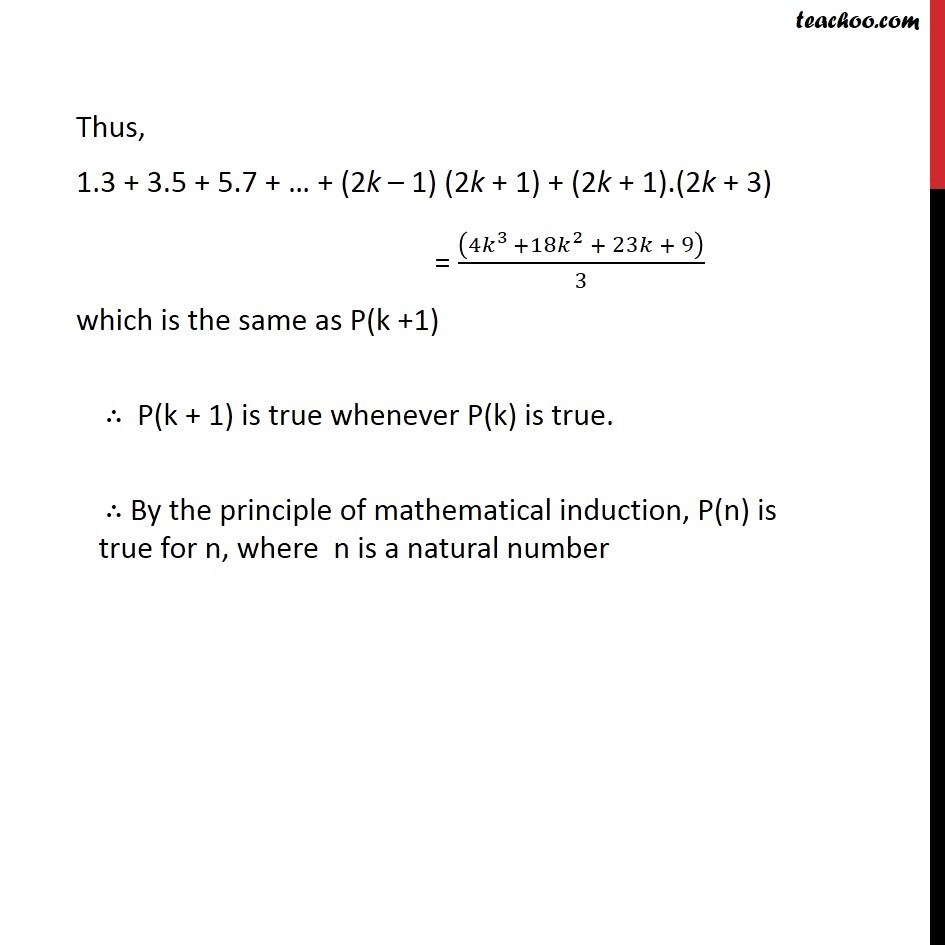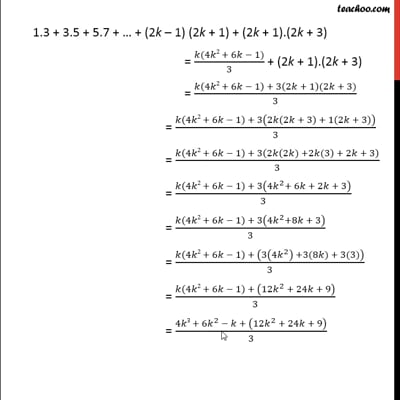This video is only available for Teachoo black users

Learn in your speed, with individual attention - Teachoo Maths 1-on-1 Class

### Transcript

Question 7: Prove the following by using the principle of mathematical induction for all n N: 1.3 + 3.5 + 5.7 + + (2n 1) (2n + 1) = ( (4 2 + 6 1))/3 Let P(n) : 1.3 + 3.5 + 5.7 + + (2n 1) (2n + 1) = ( (4 2 + 6 1))/3 For n = 1, L.H.S = 1.3 = 3 R.H.S = (1(4.12 + 6.1 1))/3 = (4 + 6 1)/3 = 9/3 = 3 L.H.S. = R.H.S P(n) is true for n = 1 Assume P(k) is true 1.3 + 3.5 + 5.7 + + (2k 1) (2k + 1) = ( (4 2 + 6 1))/3 We will prove that P(k + 1) is true. 1.3 + 3.5 + 5.7 + + (2(k + 1) 1).(2(k + 1) + 1) = ( + 1)(4( + 1)^2 + 6( + 1) 1 )/3 1.3 + 3.5 + 5.7 + + (2k + 2 1).(2k + 2 + 1) = ( + 1)(4( ^2 + 1 + 2 )+ 6 + 6 1)/3 1.3 + 3.5 + 5.7 + + (2k + 1).(2k + 3) = ( + 1)(4 ^2 +4(1) +4(2 ) + 6 + 6 1)/3 1.3 + 3.5 + 5.7 + + (2k 1) (2k + 1) + (2k + 1).(2k + 3) = ( + 1)(4 ^2 + 4 + 8 + 6 + 6 1)/3 = ( + 1)(4 ^2 +14 + 9)/3 = (( (4 ^2 +14 + 9)+ 1(4 ^2 +14 + 9)))/3 = ((4 ^3 +18 ^2 + 23 + 9))/3 Thus, P(k +1) :1.3 + 3.5 + 5.7 + + (2k 1) (2k + 1) + (2k + 1).(2k + 3) = ((4 ^3 +18 ^2 + 23 + 9))/3 We have to prove P(k+1) from P(k) i.e. (2) from (1) From (1) 1.3 + 3.5 + 5.7 + + (2k 1) (2k + 1) = ( (4 2 + 6 1))/3 Adding (2k+1).(2k+3) both sides 1.3 + 3.5 + 5.7 + + (2k 1) (2k + 1) + (2k + 1).(2k + 3) = ( (4 2 + 6 1))/3 + (2k + 1).(2k + 3) = ( (4 2 + 6 1) + 3(2 + 1)(2 + 3))/3 = ( (4 2 + 6 1) + 3(2 (2 + 3) + 1(2 + 3)))/3 = ( (4 2 + 6 1) + 3(2 (2 ) +2 (3) + 2 + 3))/3 = ( (4 2 + 6 1) + 3(4 ^2+ 6 + 2 + 3))/3 = ( (4 2 + 6 1) + 3(4 ^2+8 + 3))/3 = ( (4 2 + 6 1) + (3(4 ^2 ) +3(8 ) + 3(3)))/3 = ( (4 2 + 6 1) + (12 ^2 + 24 + 9))/3 = (4 3 + 6 ^2 + (12 ^2 + 24 + 9))/3 = (4 3 + 6 ^2 + 12 ^2 + 24 + 9)/3 = ((4 ^3 +18 ^2 + 23 + 9))/3 Thus, 1.3 + 3.5 + 5.7 + + (2k 1) (2k + 1) + (2k + 1).(2k + 3) = ((4 ^3 +18 ^2 + 23 + 9))/3 which is the same as P(k +1) P(k + 1) is true whenever P(k) is true. By the principle of mathematical induction, P(n) is true for n, where n is a natural number